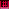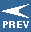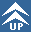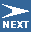Home    |    Teacher    |    Parents    |    Glossary    |    About UsEmail this page to a friendFor Parents· Help Your Kids · Family Math · Advice & Answers · Homeschooling · Special NeedsResources· Cool Tools · Formulas & Tables · References · Test Preparation · Study Tips · Wonders of MathSearchHelping Your Child Learn Math - June 1999

## What Our Children Are Learning

You probably remember studying "arithmetic"- adding, subtracting, multiplying, and dividing when you were in elementary school. Now, children are starting right away to learn about the broad ideas associated with math, including problem solving, communicating mathematically, reasoning, and number sense. Listed below are a few of the key mathematical concepts that appear in elementary school math books and classroom instruction today.Algebra: Algebra is a generalization of arithmetic in which letters of the alphabet represent numbers or a specified set of numbers, and are related by operations that hold for all numbers in the set. Children use algebra when they solve problems like 4+o = 7, with letters replacing the box as children get older.Arithmetic: Arithmetic is the knowledge and the ability to add, subtract, multiply, and divide whole numbers and fractions. For example: 5+1, 10-5, 6x2, 3÷12, and ¾ + ½.Calculus: Calculus is about how quantities change. By understanding calculus, people can use math to make predictions about things that change over time.Estimation: Estimation is the act of approximating or guessing the number value of something. We use estimation regularly to determine such things as how many, how heavy, and how full. Being a good estimator also helps children know if answers on calculators or other computer-generated data make sense.Geometry and spatial sense: Geometry begins with children recognizing shapes by their characteristics and extends to their being able to use formulas and algebra to determine important details about each shape. For example, a= ½ (bxh) is the formula for determining the area of a triangle.Measurement: Measurement is determining the lengths, areas, volume, time, and other quantities. Children need to know common units of measure such as inches or kilograms and how to use measurement tools.Number sense: Number sense is understanding the relative sizes of numbers and how to use them, whether doing arithmetic, estimation, measurement, or classification.Probability: Probability is determining the likelihood that something will happen, often expressed as a fraction or a ratio1 in 10, 1/10.Statistics: Statistics is the collection and analysis of numerical data. Taking a census and counting people is a statistical activity.[How Will Math Look in Your Child's Classroom?]